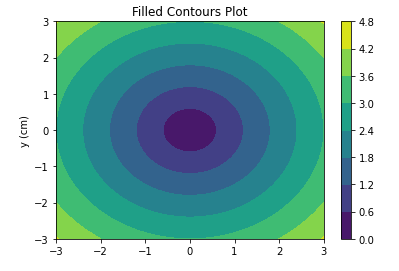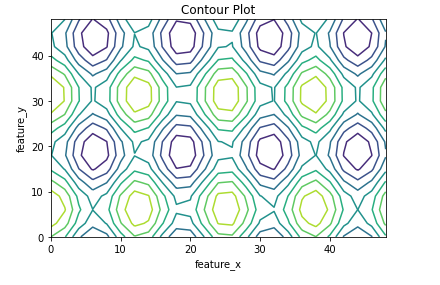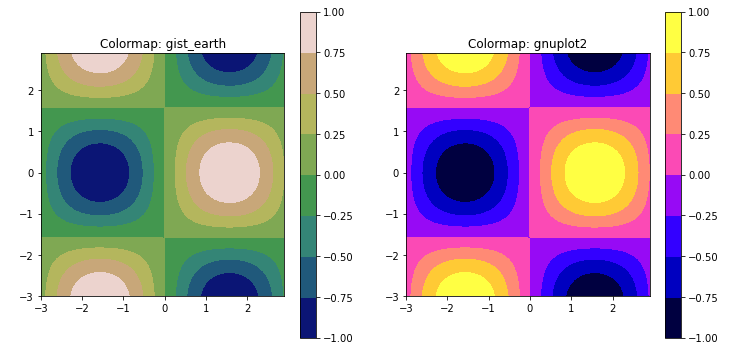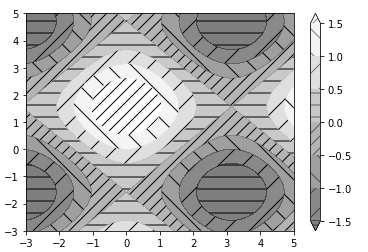# Introduction

In this tutorial, we will be learning how we can build matplotlib contour plot for your data science and machine learning projects. We will go through the syntax and will see various interesting examples of contour plots that can be built using the matplotlib library. If you are a beginner, this tutorial will be really useful for you.

### Importing Matplotlib Library

Before beginning with this matplotlib bar plot tutorial, we’ll need to import the Matplotlib Library. So let’s import Matplotlib

In :
```import matplotlib.pyplot as plt
import numpy as np
```

## Matplotlib Contour Plot Tutorial

### Contour Plot Syntax

Let’s look at the syntax of the function used for creating a contour plot in matplotlib.

contourf([X, Y,] Z, [levels], **kwargs)

• X, Y : array-like, optional – These parameters are the values for the first 2 dimensions.
• Z : array-like – The height values that are used for contour plot.
• Levels : int or array-like, optional – This parameter determines the number and positions of contour lines/regions.

### Example 1: Filled Contour Plot in Matplotlib

This example shows how to create a simple contour plot. First, we generate data for both the axes. With the help of contourf function of matplotlib we can plot a contour plot.

The plot generally takes three values i.e. X,Y,Z which are the values of three dimensions.

We will also add a color bar to the contour plot.

In :
```xlist = np.linspace(-3.0, 3.0, 100)
ylist = np.linspace(-3.0, 3.0, 100)

X, Y = np.meshgrid(xlist, ylist)
Z = np.sqrt(X**2 + Y**2)

fig,ax=plt.subplots(1,1)
cp = ax.contourf(X, Y, Z)
fig.colorbar(cp) # Add a colorbar to a plot
ax.set_title('Filled Contours Plot')

#ax.set_xlabel('x (cm)')
ax.set_ylabel('y (cm)')
plt.show()
```
Output:### Example 2: Boundary Based Non-Filled Contour Plot

For building a non-filled boundary-based contour plot, we first use arange function for generating data.

After all the three dimensions have values, the contour function is provided these values which help in plotting the contour plot.

In :
```feature_x = np.arange(0, 50, 2)
feature_y = np.arange(0, 50, 3)

# Creating 2-D grid of features
[X, Y] = np.meshgrid(feature_x, feature_y)

fig, ax = plt.subplots(1, 1)

Z = np.cos(X / 2) + np.sin(Y / 4)

# plots contour lines
ax.contour(X, Y, Z)

ax.set_title('Contour Plot')
ax.set_xlabel('feature_x')
ax.set_ylabel('feature_y')

plt.show()
```
Output:### Example 3: Pair of Contour Plot

This 3rd example talks about how to plot multiple contour plots adjacent to each other.

With the help of subplots function, these two plots are plotted. We call the contourf function for plotting these contour plots. Along with this colorbar are also plotted.

In :
```x = np.arange(-3.0, 3.0, 0.1)
y = np.arange(-3.0, 3.0, 0.1)

X, Y = np.meshgrid(x, y)

Z = np.sin(X)*np.cos(Y)

fig, (ax1, ax2) = plt.subplots(1, 2, figsize=(12,6))

mycmap1 = plt.get_cmap('cool_r')
ax1.set_aspect('equal')
ax1.set_title('Colormap: cool_r')
cf1 = ax1.contourf(X,Y,Z, cmap=mycmap1)

fig.colorbar(cf1, ax=ax1)

mycmap2 = plt.get_cmap('tab20_r')
ax2.set_aspect('equal')
ax2.set_title('Colormap: tab20_r')
cf2 = ax2.contourf(X,Y,Z, cmap=mycmap2)

fig.colorbar(cf2, ax=ax2)

plt.show()
```
Output:### Example 4: Hatched Contour Plot

This last example of the contour plot tutorial tells us about the way for building a hatched contour plot.

The data is generated for all three dimensions. The contour plot will be a 2D plot, this will lead to flattening of x and y dimensions.

To build hatches, we will be using hatches parameter for this in contourf function.

In :
```x = np.linspace(-3, 5, 150).reshape(1, -1)
y = np.linspace(-3, 5, 120).reshape(-1, 1)
z = np.cos(x) + np.sin(y)

# we no longer need x and y to be 2 dimensional, so flatten them.
x, y = x.flatten(), y.flatten()

fig1, ax1 = plt.subplots()
cs = ax1.contourf(x, y, z, hatches=['-', '/', '\\', '//'],
cmap='gray', extend='both', alpha=0.5)
fig1.colorbar(cs)
```
Output:## Conclusion

We have reached the end of this tutorial focusing on building contour plot with matplotlib. Here we learned about different types of contour plots that can be build using matplotlib and covered intricate details that should be taken care of while building them. We also looked at how we can play around with the parameters for producing a combination of different contour plots.

Reference –  Matplotlib Documentation